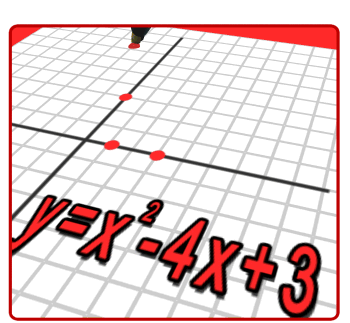•The Central Bucks School District uses the Math in Focus program as part of their curriculum .  This program is built on a framework developed by the Singapore Ministry of Education that draws on years of leading international research on effective teaching and learning of mathematics.

Singapore Math refers both to the curriculum in use in Singapore, as well as the way math is taught.  Instruction focuses on problem solving as a central skill instead of on direct instruction and memorization.  Students are required to understand not just the HOW, but the WHY of mathematical processes.  They learn the material at an increasingly deep level, resulting in their being able to apply mathematice competently and confidently.

Mathematical problem solving lies at the heart of the Singapore Math approach.  There are five key aspects to the approach. They can be summarized as Skills, Concepts, Processes, Metacognition, and Attitudes.  Students will use a Concrete-Pictorial-Abstract development of concepts using model drawings to connect visual representation to problem solving and algebra.

Third Grade focuses on the following:
• Operations and Algebraic Thinking
• Represent and solve problems involving multiplication and division.
• Understand properties of multiplication and the relationship between multiplication and division.
• Multiply and divide within 100.
• Solve problems involving the four operations, and identify and explain patterns in arithmetic.
• Number and Operations in Base Ten
• Use place value understanding and properties of operations to perform multi-digit arithmetic.
• Numbers and Operations - Fractions
• Develop understanding of fractions as numbers.
• Measurement and Data
• Solve problems involving measurement and estimation of intervals of time, liquid volumes, and masses of objects.
• Represent and interpret data.
• Geometric measurement: understand concepts of area and relate area to multiplication and to addition.
• Geometric measurement: recognize perimeter as an attritube of plane figures and distinguish between linear and area measures.
• Geometry
• Reason with shapes and their attributes.
Here is a link to games that coincide with each chapter: